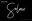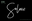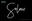"].join(""),l.id=h,(m?l:n).innerHTML+=f,n.appendChild(l),m||(n.style.background="",n.style.overflow="hidden",k=g.style.overflow,g.style.overflow="hidden",g.appendChild(n)),i=c(l,a),m?l.parentNode.removeChild(l):(n.parentNode.removeChild(n),g.style.overflow=k),!!i},x={}.hasOwnProperty,y;!B(x,"undefined")&&!B(x.call,"undefined")?y=function(a,b){return x.call(a,b)}:y=function(a,b){return b in a&&B(a.constructor.prototype[b],"undefined")},Function.prototype.bind||(Function.prototype.bind=function(b){var c=this;if(typeof c!="function")throw new TypeError;var d=u.call(arguments,1),e=function(){if(this instanceof e){var a=function(){};a.prototype=c.prototype;var f=new a,g=c.apply(f,d.concat(u.call(arguments)));return Object(g)===g?g:f}return c.apply(b,d.concat(u.call(arguments)))};return e}),q.touch=function(){var c;return"ontouchstart"in a||a.DocumentTouch&&b instanceof DocumentTouch?c=!0:w(["@media (",m.join("touch-enabled),("),h,")","{#modernizr{top:9px;position:absolute}}"].join(""),function(a){c=a.offsetTop===9}),c},q.csstransforms=function(){return!!F("transform")},q.csstransforms3d=function(){var a=!!F("perspective");return a&&"webkitPerspective"in g.style&&w("@media (transform-3d),(-webkit-transform-3d){#modernizr{left:9px;position:absolute;height:3px;}}",function(b,c){a=b.offsetLeft===9&&b.offsetHeight===3}),a},q.fontface=function(){var a;return w('@font-face {font-family:"font";src:url("https://")}',function(c,d){var e=b.getElementById("smodernizr"),f=e.sheet||e.styleSheet,g=f?f.cssRules&&f.cssRules?f.cssRules.cssText:f.cssText||"":"";a=/src/i.test(g)&&g.indexOf(d.split(" "))===0}),a};for(var G in q)y(q,G)&&(v=G.toLowerCase(),e[v]=q[G](),t.push((e[v]?"":"no-")+v));return e.addTest=function(a,b){if(typeof a=="object")for(var d in a)y(a,d)&&e.addTest(d,a[d]);else{a=a.toLowerCase();if(e[a]!==c)return e;b=typeof b=="function"?b():b,typeof f!="undefined"&&f&&(g.className+=" supports-"+(b?"":"no-")+a),e[a]=b}return e},z(""),i=k=null,e._version=d,e._prefixes=m,e._domPrefixes=p,e._cssomPrefixes=o,e.testProp=function(a){return D([a])},e.testAllProps=F,e.testStyles=w,g.className=g.className.replace(/(^|\s)no-js(\s|\$)/,"\$1\$2")+(f?" supports-js supports-"+t.join(" supports-"):""),e}(this,this.document); /*! * enquire.js v2.1.2 - Awesome Media Queries in JavaScript * Copyright (c) 2014 Nick Williams - http://wicky.nillia.ms/enquire.js * License: MIT (http://www.opensource.org/licenses/mit-license.php) */ !function(a,b,c){var d=window.matchMedia;"undefined"!=typeof module&&module.exports?module.exports=c(d):"function"==typeof define&&define.amd?define(function(){return b[a]=c(d)}):b[a]=c(d)}("enquire",this,function(a){"use strict";function b(a,b){var c,d=0,e=a.length;for(d;e>d&&(c=b(a[d],d),c!==!1);d++);}function c(a){return"[object Array]"===Object.prototype.toString.apply(a)}function d(a){return"function"==typeof a}function e(a){this.options=a,!a.deferSetup&&this.setup()}function f(b,c){this.query=b,this.isUnconditional=c,this.handlers=[],this.mql=a(b);var d=this;this.listener=function(a){d.mql=a,d.assess()},this.mql.addListener(this.listener)}function g(){if(!a)throw new Error("matchMedia not present, legacy browsers require a polyfill");this.queries={},this.browserIsIncapable=!a("only all").matches}return e.prototype={setup:function(){this.options.setup&&this.options.setup(),this.initialised=!0},on:function(){!this.initialised&&this.setup(),this.options.match&&this.options.match()},off:function(){this.options.unmatch&&this.options.unmatch()},destroy:function(){this.options.destroy?this.options.destroy():this.off()},equals:function(a){return this.options===a||this.options.match===a}},f.prototype={addHandler:function(a){var b=new e(a);this.handlers.push(b),this.matches()&&b.on()},removeHandler:function(a){var c=this.handlers;b(c,function(b,d){return b.equals(a)?(b.destroy(),!c.splice(d,1)):void 0})},matches:function(){return this.mql.matches||this.isUnconditional},clear:function(){b(this.handlers,function(a){a.destroy()}),this.mql.removeListener(this.listener),this.handlers.length=0},assess:function(){var a=this.matches()?"on":"off";b(this.handlers,function(b){b[a]()})}},g.prototype={register:function(a,e,g){var h=this.queries,i=g&&this.browserIsIncapable;return h[a]||(h[a]=new f(a,i)),d(e)&&(e={match:e}),c(e)||(e=[e]),b(e,function(b){d(b)&&(b={match:b}),h[a].addHandler(b)}),this},unregister:function(a,b){var c=this.queries[a];return c&&(b?c.removeHandler(b):(c.clear(),delete this.queries[a])),this}},new g}); /*! * imagesLoaded PACKAGED v4.1.0 * JavaScript is all like "You images are done yet or what?" * MIT License */ !function(t,e){"function"==typeof define&&define.amd?define("ev-emitter/ev-emitter",e):"object"==typeof module&&module.exports?module.exports=e():t.EvEmitter=e()}(this,function(){function t(){}var e=t.prototype;return e.on=function(t,e){if(t&&e){var i=this._events=this._events||{},n=i[t]=i[t]||[];return-1==n.indexOf(e)&&n.push(e),this}},e.once=function(t,e){if(t&&e){this.on(t,e);var i=this._onceEvents=this._onceEvents||{},n=i[t]=i[t]||[];return n[e]=!0,this}},e.off=function(t,e){var i=this._events&&this._events[t];if(i&&i.length){var n=i.indexOf(e);return-1!=n&&i.splice(n,1),this}},e.emitEvent=function(t,e){var i=this._events&&this._events[t];if(i&&i.length){var n=0,o=i[n];e=e||[];for(var r=this._onceEvents&&this._onceEvents[t];o;){var s=r&&r[o];s&&(this.off(t,o),delete r[o]),o.apply(this,e),n+=s?0:1,o=i[n]}return this}},t}),function(t,e){"use strict";"function"==typeof define&&define.amd?define(["ev-emitter/ev-emitter"],function(i){return e(t,i)}):"object"==typeof module&&module.exports?module.exports=e(t,require("ev-emitter")):t.imagesLoaded=e(t,t.EvEmitter)}(window,function(t,e){function i(t,e){for(var i in e)t[i]=e[i];return t}function n(t){var e=[];if(Array.isArray(t))e=t;else if("number"==typeof t.length)for(var i=0;i
Shopping Cart
FREE SHIPPING ON ALL ORDERS# Solrae Gift Card

## \$10.00Best PricesFree ShippingPETA Cruelty-Free and Vegan

You have great taste {duh!}, what a perfect gift - a Solrae gift card. Perfect for your loved one {or yourself - no judging}. The recipient will be smiling big when they see their perfectly bronzed face!

Gift cards are delivered by email and contain instructions to redeem them at checkout

*GIFT CARDS PURCHASED ONLINE ARE ONLY VALID FOR ONLINE PURCHASES

## Customer Reviews

Based on 1 review
100%
(1)
0%
(0)
0%
(0)
0%
(0)
0%
(0)
A
Alex Piccolomini